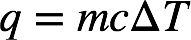# Problem: The temperature of the cooling water as it leaves the hot engine of an automobile is 240°F. After it passes through the radiator it has a temperature of 175°F. Calculate the amount of heat transferred from the engine to the surroundings by one gallon of water with a specific heat of 4.184 J/g°C.

###### FREE Expert Solution

Recall: The amount of heat can be calculated using the following formula:where m = mass of the object (in grams), c = specific heat (in J/g • ˚C), ΔT = change in temperature (in ˚C).

The initial temperature is 240 ˚F and the final temperature is 175 ˚F. Recall that ˚F = 1.8 (˚C) + 32. Converting the given temperatures to ˚C:

83% (362 ratings)###### Problem Details

The temperature of the cooling water as it leaves the hot engine of an automobile is 240°F. After it passes through the radiator it has a temperature of 175°F. Calculate the amount of heat transferred from the engine to the surroundings by one gallon of water with a specific heat of 4.184 J/g°C.